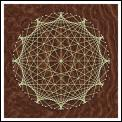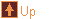String Art 15 images tree :: Geometry > String Art
In This Album:

 Image List Thumbnail Page
These string art images illustrate various curves, epicycloids and hypocycloids, being described as the envelope of set of lines.

The methods of construction are given in a chapter on curves in Geometry and the Visual Arts by Dan Pedoe.

The images have been produced from the following povray files - string1.pov, string2.pov, string3.pov.

 Image List

Cardioid  Med    Lrg
 Description : The cardioid is the path followed by a point on the circumference of a circle of radius one unit as it rolls around the circumference of another circle of radius one unit. It is formed here from its tangents by laying N points equally on a circle, and joining point n to the point (2n + N/2) mod N.Nephroid  Med    Lrg
 Description : The nephroid is the path followed by a point on the circumference of a circle of radius one unit as it rolls around the circumference of a circle of radius two units. It is formed here from its tangents by laying N points equally on a circle, and joining point n to the point (3n + N/2) mod N.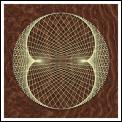Epicycloid 1:3  Med    Lrg
 Description : The curve is the path followed by a point on the circumference of a circle of radius one unit as it rolls around the circumference of a circle of radius three units. It is formed here from its tangents by laying N points equally on a circle, and joining point n to the point (4n + N/2) mod N.Epicycloid 1:4  Med    Lrg
 Description : The curve is the path followed by a point on the circumference of a circle of radius one unit as it rolls around the circumference of a circle of radius four units. It is formed here from its tangents by laying N points equally on a circle, and joining point n to the point (5n + N/2) mod N.Epicycloid 1:5  Med    Lrg
 Description : The curve is the path followed by a point on the circumference of a circle of radius one unit as it rolls around the circumference of a circle of radius five units. It is formed here from its tangents by laying N points equally on a circle, and joining point n to the point (6n + N/2) mod N.Epicycloid 1:6  Med    Lrg
 Description : The curve is the path followed by a point on the circumference of a circle of radius one unit as it rolls around the circumference of a circle of radius six units. It is formed here from its tangents by laying N points equally on a circle, and joining point n to the point (7n + N/2) mod N.Epicycloid 1:8  Med    Lrg
 Description : The curve is the path followed by a point on the circumference of a circle of radius one unit as it rolls around the circumference of a circle of radius eight units. It is formed here from its tangents by laying N points equally on a circle, and joining point n to the point (9n + N/2) mod N.Epicycloid 1:12  Med    Lrg
 Description : The curve is the path followed by a point on the circumference of a circle of radius one unit as it rolls around the circumference of a circle of radius twelve units. It is formed here from its tangents by laying N points equally on a circle, and joining point n to the point (13n + N/2) mod N.Astroid  Med    Lrg
 Description : The astroid is the path followed by a point on the circumference of a circle of radius one unit as it rolls inside the circumference of a circle of radius four units. It is formed here from its tangents by choosing a length L and then joining points on the x-axis to points at distance L on the y-axis. All the tangent strings in the image have length L.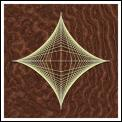Deltoid  Med    Lrg
 Description : The deltoid is the path followed by a point on the circumference of a circle of radius one unit as it rolls inside the circumference of a circle of radius three units. It is formed here from its tangents by laying N points equally on a circle (the inner circle), and joining point n to the point (N/2 - 2n) mod N. The strings are extended to an outer circle whose radius is 3 times the inner circle radius.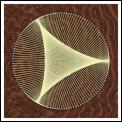Astroid  Med    Lrg
 Description : The astroid is the path followed by a point on the circumference of a circle of radius one unit as it rolls inside the circumference of a circle of radius four units. It is formed here from its tangents by laying N points equally on a circle (the inner circle), and joining point n to the point (N/2 - 3n) mod N. The strings are extended to an outer circle whose radius is 2 times the inner circle radius.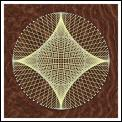Hypocycloid 1:5  Med    Lrg
 Description : The curve is the path followed by a point on the circumference of a circle of radius one unit as it rolls inside the circumference of a circle of radius five units. It is formed here from its tangents by laying N points equally on a circle (the inner circle), and joining point n to the point (N/2 - 4n) mod N. The strings are extended to an outer circle whose radius is 5/3 times the inner circle radius.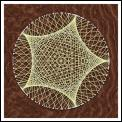Hypocycloid 1:6  Med    Lrg
 Description : The curve is the path followed by a point on the circumference of a circle of radius one unit as it rolls inside the circumference of a circle of radius six units. It is formed here from its tangents by laying N points equally on a circle (the inner circle), and joining point n to the point (N/2 - 5n) mod N. The strings are extended to an outer circle whose radius is 3/2 times the inner circle radius.Hypocycloid 1:8  Med    Lrg
 Description : The curve is the path followed by a point on the circumference of a circle of radius one unit as it rolls inside the circumference of a circle of radius eight units. It is formed here from its tangents by laying N points equally on a circle (the inner circle), and joining point n to the point (N/2 - 7n) mod N. The strings are extended to an outer circle whose radius is 4/3 times the inner circle radius.Hypocycloid 1:12  Med    Lrg
 Description : The curve is the path followed by a point on the circumference of a circle of radius one unit as it rolls inside the circumference of a circle of radius twelve units. It is formed here from its tangents by laying N points equally on a circle (the inner circle), and joining point n to the point (N/2 - 11n) mod N. The strings are extended to an outer circle whose radius is 6/5 times the inner circle radius.